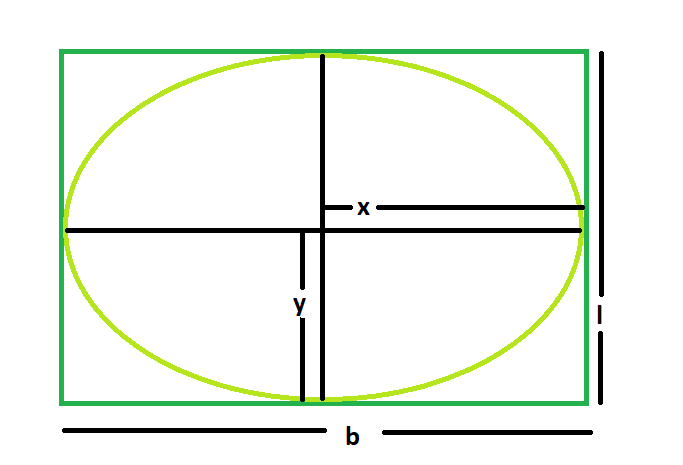GeeksforGeeks App
Open AppBrowser
Continue

# Area of the biggest ellipse inscribed within a rectangle

Given here is a rectangle of length l & breadth b, the task is to find the area of the biggest ellipse that can be inscribed within it.
Examples:

```Input: l = 5, b = 3
Output: 11.775

Input: 7, b = 4
Output: 21.98```Approach

1. Let, the length of the major axis of the ellipse = 2x and the length of the minor axis of the ellipse = 2y
2. From the diagram, it is very clear that,
2x = l
2y = b

3. So, Area of the ellipse = (π * x * y) = (π * l * b) / 4

Below is the implementation of the above approach:

## C++

 `// C++ Program to find the biggest ellipse``// which can be inscribed within the rectangle` `#include ``using` `namespace` `std;` `// Function to find the area``// of the ellipse``float` `ellipse(``float` `l, ``float` `b)``{` `    ``// The sides cannot be negative``    ``if` `(l < 0 || b < 0)``        ``return` `-1;` `    ``// Area of the ellipse``    ``float` `x = (3.14 * l * b) / 4;` `    ``return` `x;``}` `// Driver code``int` `main()``{``    ``float` `l = 5, b = 3;``    ``cout << ellipse(l, b) << endl;` `    ``return` `0;``}`

## Java

 `// Java Program to find the biggest rectangle``// which can be inscribed within the ellipse``import` `java.util.*;``import` `java.lang.*;``import` `java.io.*;` `class` `GFG``{``    ` `// Function to find the area``// of the rectangle``static` `float` `ellipse(``float` `l, ``float` `b)``{``    ` `    ``// a and b cannot be negative``    ``if` `(l < ``0` `|| b < ``0``)``        ``return` `-``1``;``    ``float` `x = (``float``)(``3.14` `* l * b) / ``4``;` `    ``return` `x;``    ` `}``    ` `// Driver code``public` `static` `void` `main(String args[])``{``    ``float` `a = ``5``, b = ``3``;``    ``System.out.println(ellipse(a, b));``}``}` `// This code is contributed``// by Mohit Kumar`

## Python3

 `# Python3 Program to find the biggest ellipse``# which can be inscribed within the rectangle` `# Function to find the area``# of the ellipse``def` `ellipse(l, b):` `    ``# The sides cannot be negative``    ``if` `l < ``0` `or` `b < ``0``:``        ``return` `-``1` `    ``# Area of the ellipse``    ``x ``=` `(``3.14` `*` `l ``*` `b) ``/` `4` `    ``return` `x` `# Driver code``if` `__name__ ``=``=` `"__main__"``:` `    ``l, b ``=` `5``, ``3``    ``print``(ellipse(l, b))` `# This code is contributed``# by Rituraj Jain`

## C#

 `// C# Program to find the biggest rectangle``// which can be inscribed within the ellipse``using` `System;` `class` `GFG``{``    ` `// Function to find the area``// of the rectangle``static` `float` `ellipse(``float` `l, ``float` `b)``{``    ` `    ``// a and b cannot be negative``    ``if` `(l < 0 || b < 0)``        ``return` `-1;``    ``float` `x = (``float``)(3.14 * l * b) / 4;` `    ``return` `x;``    ` `}``    ` `// Driver code``public` `static` `void` `Main()``{``    ``float` `a = 5, b = 3;``    ``Console.WriteLine(ellipse(a, b));``}``}` `// This code is contributed``// by Code_Mech.`

## PHP

 ``

## Javascript

 ``

Output:

`11.775`

Time Complexity: O(1)

Auxiliary Space: O(1)

My Personal Notes arrow_drop_up